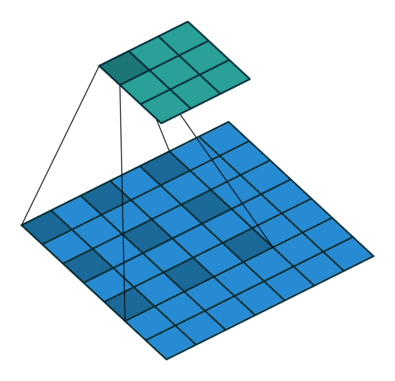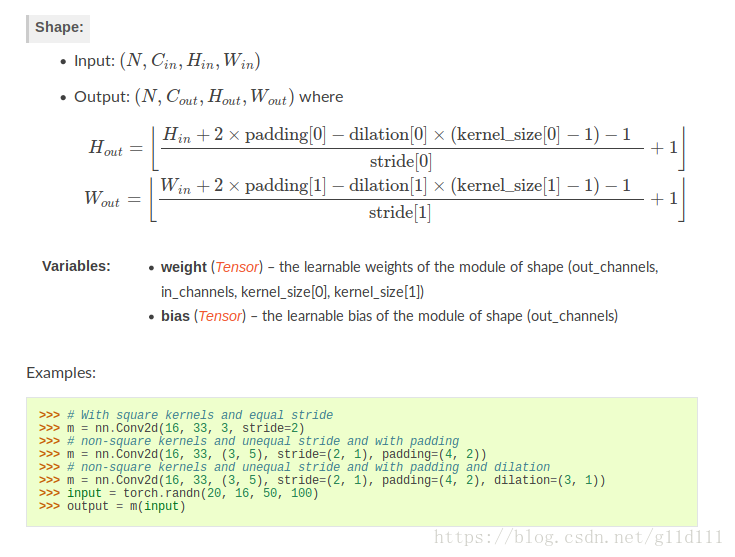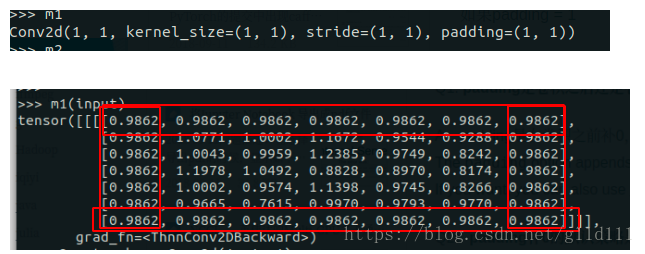• Pytorch 从0开始学（6）——Conv2d 详解
2021-01-26 20:37:24

您是否在使用Conv2d时遇见问题了呢？

您是否还在以Conv2d(128， 256， 3)的方式简单使用这个最具魅力的layer呢？

想更了解Conv2d么？让我们一起来深入看看它的真容吧，让我们触到它更高端的用法。

在第5节中，我们已经使用了Linear层来构建模型。其来自于torch.nn中

首先，我们先对torch.nn 来个整体的认识。官方链接：

可以看到，在torch.nn下包含了我们模型的概念、一些常用层、损失函数等的定义。我们不一一详细说，只说我们刚开始操作时最经常用到的。今天我们从最常用的2维卷积层说起。

首先还是看官方文档对Conv2d的整体定义：

Conv2d，就是用来实现2d卷积操作的。要使用好这个类，我们先得搞清楚它的输入参数都是些啥？

in_channels —— 输入的channels数

out_channels —— 输出的channels数

kernel_size ——卷积核的尺寸，可以是方形卷积核、也可以不是，下边example可以看到

stride —— 步长，用来控制卷积核移动间隔

bias ——是否使用偏置(即out = wx+b中的b)

以上参数，均是大家了解相对较多的参数项目。

dilation —— 这个参数简单说，设定了取数之间的间隔，下图可表达其意思

dilation = 1

dilation = 2

上图中蓝色为选取进行卷积计算的值。通过对比，即可了解该选项的作用

groups —— 进行分组卷积的组数

这个参数的使用不太好理解，首先还是贴原文档的说明

这个其实是将输入和输出进行分组，然后进行对应的映射，最后连接形成输出。引网络上的一张图，大概可以看出此参数的作用。引至：

参数的输入数据格式如下：

以上对参数进行了较为详尽的说明，基本上可以使用了！

官方给出了几个例子

1)方形卷积核、行列相同步长(With square kernels and equal stride)

m = nn.Conv2d(16, 33, 3, stride=2)

2)非方形卷积核、行列采用不同步长，并进行扩边

m = nn.Conv2d(16, 33, (3, 5), stride=(2, 1), padding=(4, 2))

3)非方形卷积核、行列采用不同步长、数据采用稀疏，并进行扩边

m = nn.Conv2d(16, 33, (3, 5), stride=(2, 1), padding=(4, 2), dilation=(3, 1))

通过以上例子，可知使用Conv2d的使用。

最后，我们看下Conv2d的源码，通过底层看出其实现：

该类的源码定义中，我们可以看到其中定义了3个函数：__init__、forward和conv2d_forward。__init__中可以看到，对相关参数进行了默认初始化；而在forward中，表明了这个底层实现函数是采用的nn.functional.conv2d来实现。

以上，就对该类的使用进行了一次梳理，希望大家使用起来更加顺畅！

【备注】

由于也是刚转战pytorch，有错误的地方，望各位大佬指正。也希望有兴趣一起学习的朋友一起加入进来

更多相关内容
• pytorch 的二维卷积 torch.Conv2d，宽高计算公式如下所示（假设宽高相同，这里只讨论宽的公式，高的公式类似）： Wout=Win+2×padding−dilation×(kernelsize−1)−1stride+1 W_{out} = \frac{W_{in} + 2 \times \...

pytorch 的二维卷积 torch.Conv2d，宽高计算公式如下所示（假设宽高相同，这里只讨论宽的公式，高的公式类似）：

W o u t = W i n + 2 × p a d d i n g − d i l a t i o n × ( k e r n e l s i z e − 1 ) − 1 s t r i d e + 1 W_{out} = \frac{W_{in} + 2 \times \mathrm{padding} - \mathrm{dilation} \times (\mathrm{kernelsize}-1)-1}{\mathrm{stride}} + 1

其中 dilation 为膨胀系数，kernel size 为 卷积核大小，一个膨胀系数为 2 的效果如下动图所示：由此可见，一个膨胀系数为 d d 的卷积核的感受野范围是 d × ( k s − 1 ) + 1 \mathrm{d} \times (\mathrm{ks} -1) + 1 ，例如一个膨胀系数为 2 的 3 × 3 3 \times 3 卷积核，其感受野范围是 5 × 5 （ 2 ∗ 2 + 1 ） 5 \times 5（2 * 2 + 1） 。所以感受野宽度为 W r f = d i l a t i o n × ( k e r n e l s i z e − 1 ) + 1 W_\mathrm{rf}=\mathrm{dilation} \times (\mathrm{kernelsize}-1)+1 。上述宽高公式变形后为
W o u t = W i n + 2 × p a d d i n g − ( d i l a t i o n × ( k e r n e l s i z e − 1 ) + 1 ) s t r i d e + 1 = W i n + 2 × p a d d i n g − W r f s t r i d e + 1 \begin{aligned} W_{out} &= \frac{W_{in} + 2 \times \mathrm{padding} - (\mathrm{dilation} \times (\mathrm{kernelsize}-1)+1)}{\mathrm{stride}} + 1 \\ &=\frac{W_{in} + 2 \times \mathrm{padding} - W_\mathrm{rf}}{\mathrm{stride}} + 1 \end{aligned}

当膨胀系数 d = 1 d=1 时，感受野宽度 W r f = k e r n e l s i z e W_\mathrm{rf}=\mathrm{kernelsize} ，当 p a d d i n g = 0 \mathrm{padding} = 0 时，上述式子为
W o u t = W i n − k e r n e l s i z e s t r i d e + 1 W_{out} = \frac{W_{in} - \mathrm{kernelsize}}{\mathrm{stride}} + 1

默认参数 stride=1, padding=0, dilation=1 下的公式为
W o u t = W i n − k e r n e l s i z e + 1 W_{out}=W_{in}-\mathrm{kernelsize} + 1

展开全文• 卷积函数tf.nn.conv2d1. tf.nn.conv2d在TensorFlow 的程序中加入卷积层是非常容易的。最常用的方法是 tf.nn.conv2d方法，用于在计算图中加入2D 卷积算子。简单的用法如下：tf.nn.conv2d(input, filter, strides, ...

卷积函数tf.nn.conv2d

1. tf.nn.conv2d

在TensorFlow 的程序中加入卷积层是非常容易的。最常用的方法是 tf.nn.conv2d方法，用于在计算图中加入2D 卷积算子。简单的用法如下：

tf.nn.conv2d(input, filter, strides, padding, use_cudnn_on_gpu=None, data_format=None,name=None)

2. 参数

除去name参数用以指定该操作的name，与方法有关的一共五个参数：

第一个参数input：需要做卷积的输入图像，它要求是一个Tensor，具有[batch, in_height, in_width, in_channels] 这样的shape，具体含义是 [训练时一个batch的图片数量, 图片高度, 图片宽度, 图像通道数]，注意这是一个4维的Tensor，要求类型为float32和float64其中之一

第二个参数filter：卷积核大小，它要求是一个Tensor，具有[filter_height, filter_width, in_channels, out_channels]这样的shape，具体含义是[卷积核的高度，卷积核的宽度，图像通道数，卷积核个数]，要求类型与参数input相同,filter的通道数要求与input的in_channels一致，有一个地方需要注意，第三维in_channels，就是参数input的第四维

第三个参数strides：表示步长，卷积时在图像每一维的步长，这是一个一维的向量，长度4，strides=strides=1

第五个参数：use_cudnn_on_gpu:bool类型，是否使用cudnn加速，默认为true

data_format: string类型的量 “NHWC”, “NCHW”， 默认为”NHWC”。指定输入输出数据格式，默认格式为”NHWC”, 数据按这样的顺序存储： [batch, in_height, in_width, in_channels],也可以用这种方式：”NCHW”, 数据按这样的顺序存储： [batch, in_channels, in_height, in_width]

结果返回一个Tensor，这个输出，就是我们常说的feature map,type与input相同

当卷积核与图像重叠时，它应当落在图像的边界内。有时，两者尺寸可能不匹配，一种较好的补救策略是对图像缺失的区域进行填充，即边界填充。

TensorFlow会用0进行边界填充，或当卷积核与图像尺寸不匹配，但又不允许卷积核跨越图像边界时，会引发一个错误。

·SAME：卷积输出与输入的尺寸相同。这里在计算如何跨越图像时，并不考虑滤波器的尺寸。选用该设置时，缺失的像素将用0填充，卷积核扫过的像素数将超过图像的实际像素数。

·VALID：在计算卷积核如何在图像上跨越时，需要考虑滤波器的尺寸。这会使卷积核尽量不越过图像的边界。在某些情形下，可能边界也会被填充。

在计算卷积时，最好能够考虑图像的尺寸，如果边界填充是必要的，则TensorFlow会有一些内置选项。在大多数比较简单的情形下，SAME都是一个不错的选择。当指定跨度参数后，如果输入和卷积核能够很好地工作，则推荐使用VALID。关于这两个参数的更多介绍，请参考

4. 数据格式data_format

tf.nn.conv2d文档详细解释了如何修改data_format(数据格式)，以使input、kernel和strides遵循某种与到目前为止所使用的格式不同的格式。

如果有某个输入张量未遵循[batch_size，height，width，channel]标准，则修改该格式便非常有用。除了修改输入的格式，使之与标准匹配外，也可修改data_format参数以使用一种不同的布局。

data_format：该参数可取为“NHWC”或“NCHW”，默认值为“NHWC”，用于指定输入和输出数据的格式。

当取默认格式“NHWC”时，数据的存储顺序为[batch，in_height，in_width，in_channels]。

若该参数取为“NCHW”，数据存储顺序为[batch，in_channels，in_height，in_width]。

数据格式定义：

N 批数据中的张量数目．即batch_size

H 每个批数据中张量的高度

W 每个批数据中张量的宽度

C 每个批数据中张量的通道数

技术交流学习，请加QQ微信：631531977

展开全文注意事项：展开全文• 这个问题在互联网上以各种形式被问到，而且有一个简单的答案，常常被忽略或混淆：简单回答：Keras Conv2D层在多通道输入(例如彩色图像)的情况下，将在所有颜色通道上应用滤波器，并将结果求和，生成等效于单色卷积...
• class torch.nn.Conv2d(in_channels, out_channels, kernel_size, stride=1, padding=0, dilation=1, groups=1, bias=True) 对由多个特征平面组成的输入信号进行2D的卷积操作。详解 torch.nn.functional.conv2d...
• 卷积函数是卷积神经...tf.nn.conv2d(input, filter, strides, padding, use_cudnn_on_gpu=None, name=None)：在给定4维的输入和过滤器的张量时，计算一个2维卷积。参数详解：input：输入的参数或者说是图像tenors...
• In other words, while the output shape of tf.layers.conv2d() is divided by the stride, the output shape of tf.layers.conv2d_transpose() is multiplied by it: # for tf.layers.conv2d_transpose() with ...
• Conv2D卷积、Conv2DTranspose 逆卷积 - 输出计算公式一、Conv2D卷积输出计算二、Conv2DTranspose 逆卷积输出计算 Conv2D卷积： 用于缩小图像的尺寸、或增加复杂度 Conv2DTranspose 逆卷积： 用于扩大图像的尺寸 ...卷积
• 想必大家最早接触卷积的概念就是在初高中的数学当中，它是一个这样的公式； (g∗h)(x)=∫−∞∞g(τ)⋅h(x−τ)dτ(g\ast h)(x)=\int_{-\infty }^{\infty }g(\tau )\cdot h(x-\tau )d\tau (g∗h)(x)=∫−∞∞​g(τ)...卷积神经网络 深度学习 卷积
• 深度学习卷积操作的维度计算(PyTorch/Tensorflow等框架中Conv1d、Conv2d和Conv3d介绍)标签：#PyTorch##卷积神经网络##深度学习#时间：2019/05/31 20:27:07作者：小木#### 一、卷积操作的维度计算卷积操作的维度计算...
• 代码：基于pytorch的验证 #%% import torch from torch import nn #%% 产生数据 N = 2 Cin =2 ...arry = torch.arange(N*Cin*4*4,dtype=torch.float32).view([N,Cin...conv1 = nn.Conv2d(in_channels=Cin,out_channe...深度学习
• ## PyTorch：学习conv1D,conv2D和conv3D

千次阅读 多人点赞 2020-03-06 18:16:10
背景 CNN是深度学习的重中之重，而conv1D，conv2D，和conv3D又是CNN的核心，所以理解conv的工作原理就变得尤为重要。在本博客中，将简单梳理一下这三种卷积，以及在PyTorch中的具体实现。 参考 ...
• 而torch.nn.Conv2d()不同，其padding超参可以为0，1，2，计算较为麻烦，原始计算公式我就不放了（因为一般dilation =1），简化公式如下： out_size = （in_size - K + 2P）/ S +1 其中，K为kernel_size的设置值，P...tensorflow pytorch 深度学习
• ## torch.nn.Conv2d详解

千次阅读 多人点赞 2020-11-22 11:30:42
class Conv2d(_ConvNd): # 初始化函数，这里主要了解有哪些参数传进来就可以了 def __init__( self, in_channels: int, out_channels: int, kernel_size: _size_2_t, stride: _size_2_t = 1, padding: _size...pytorch 卷积神经网络
• 关于 Pytorch 的CLASS torch.nn.Conv2d(in_channels, out_channels, kernel_size, stride=1, padding=0, dilation=1, groups=1, bias=True)假设 Conv2d 的输入 input 尺寸为 $(N, C_{in}, H_{in}, W_{in})$，输出 ...batch norm
• nn.Conv2d class torch.nn.ConvTranspose2d(in_channels, out_channels, kernel_size, stride=1, padding=0, output_padding=0, groups=1, bias=True) nn.Conv2d的功能是：对由多个输入平面组成的输入信号进行二维...
• pytorch的Conv2d参数详解 torch.nn.Conv2d(in_channels, out_channels, kernel_size, stride=1, padding=0, dilation=1, groups=1, bias=True, padding_mode='zeros', device=None, dtype=None) in_channels：输入...pytorch 深度学习 python
• import torchvision from torch import nn from torch.nn import Conv2d from torch.utils.data import DataLoader dataset = torchvision.datasets.CIFAR10("./dataset", False, transform=torchvision.transforms...神经网络 深度学习
• 在CNN中，卷积层（CONV）使用过滤器执行卷积操作。因为它扫描输入图像的尺寸。它的超参数包括滤波器大小，可以是2x2、3x3、4x4、5x5（或其它）和步长S。结果输出O称为特征映射或激活映射，具有使用输入层计算的所有...pytorch
• pytorch conv2d padding 的填充策略padding数值的意义 先回顾一下卷积公式。假设33的卷积核，那么填充后的结果就是y=x1k1+x2k2+…+x9k9. 如果有bias的话 ，那y=x1k1+x2k2+…+x9k9 + bias 。 如果卷积核大小为1，那么...python c++ phppytorch maxpool2d
• nn.Conv2d和nn.ConvTranspose2d参数说明及区别 1、nn.Conv2d(in_channels，out_channels，kernel_size，stride=1，padding=0，dilation=1，groups=1，bias=True) in_channels：输入维度 out_channels：输出维度 ...
• nn.Conv2d:常用在图像 (B,C,H,W) (batch,channel,height,width) （批数量，通道数，高度，长度） nn.Conv3d:常用在（时序）帧图像 (B,C,D,H,W) (batch,channel,depth,height,width) （批...
• nn.conv2d(256, 256, 3, 3) 假设输入大小为[3, 128, 128],输出的大小则为128-3+1=126，这一层参数个数为256*3*3+256=2560. 假设输入尺寸为[C_in, H, W]，则输出...nn.conv2d(C_in, C_out, K, K)参数个数的计算公式为C_
• PyTorch学习笔记：nn.Conv2d——二维卷积运算解读nn.Conv2d——二维卷积运算代码案例一般用法输出卷积运算的参数填充方式零填充镜像填充复制填充循环填充官方文档 nn.Conv2d——二维卷积运算 torch.nn.Conv2d(in_...pytorch 深度学习 python
• tf.layers.conv2d_transpose如下图所示，蓝色为输入，绿色输出，灰色为卷积核，虚线网格为0，所以也可以看作是补０之后的正常卷积 　 tf.layers.conv2d_transpose(inputs=img, filters=16, kernel_size=(6, 6), ...反卷积 空洞卷积...